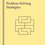# INMO 1992

This is the first question of the 1992 INMO (Indian National Maths Olympiad). People have essentially cursed me for doing this geometry problem by bashing. It has a plain geometric proof which is way more prettier than mine. But still, here's the question and my solution:

A triangle ABC has $\angle B$ half of $\angle A$. Prove that $a=b(b+c)$.

Proof: First take $\angle A=2x$. So, $\angle B=x$.

By sine rule, $\frac{a}{\sin2x}$=$\frac{b}{sinx}$=$\frac{c}{\sin3x}$. From here you can get b and c in terms of a (and some trigonometric functions) and you'll be done. This proof requires considerably less geometric knowledge and basic algebraic manipulation, though one could argue that this destroys the beauty of the problem. Comments are extremely welcome.Note by Rajdeep Ghosh
3 years, 10 months ago

This discussion board is a place to discuss our Daily Challenges and the math and science related to those challenges. Explanations are more than just a solution — they should explain the steps and thinking strategies that you used to obtain the solution. Comments should further the discussion of math and science.

When posting on Brilliant:

• Use the emojis to react to an explanation, whether you're congratulating a job well done , or just really confused .
• Ask specific questions about the challenge or the steps in somebody's explanation. Well-posed questions can add a lot to the discussion, but posting "I don't understand!" doesn't help anyone.
• Try to contribute something new to the discussion, whether it is an extension, generalization or other idea related to the challenge.

MarkdownAppears as
*italics* or _italics_ italics
**bold** or __bold__ bold
- bulleted- list
• bulleted
• list
1. numbered2. list
1. numbered
2. list
Note: you must add a full line of space before and after lists for them to show up correctly
paragraph 1paragraph 2

paragraph 1

paragraph 2

[example link](https://brilliant.org)example link
> This is a quote
This is a quote
    # I indented these lines
# 4 spaces, and now they show
# up as a code block.

print "hello world"
# I indented these lines
# 4 spaces, and now they show
# up as a code block.

print "hello world"
MathAppears as
Remember to wrap math in $$ ... $$ or $ ... $ to ensure proper formatting.
2 \times 3 $2 \times 3$
2^{34} $2^{34}$
a_{i-1} $a_{i-1}$
\frac{2}{3} $\frac{2}{3}$
\sqrt{2} $\sqrt{2}$
\sum_{i=1}^3 $\sum_{i=1}^3$
\sin \theta $\sin \theta$
\boxed{123} $\boxed{123}$

Sort by:

doing the problem is the main thing whether u bash or use plain geometry.but I had done it using plain geometry with the help of angular bisector theorem.

- 3 years, 10 months ago

- 3 years, 9 months ago

The only beauty of maths is to solve it in shorter and easier steps.

- 3 years, 4 months ago